# 2.2 Modules and Clients

Each program that you have composed so far consists of Python code that resides in a single .py file. For large programs, keeping all the code in a single file is restrictive and unnecessary. Fortunately, it is easy in Python to call a function that is defined in another file. This ability has two important consequences:

• It enables code reuse. One program can use code that is already written and debugged, not by copying the code, but just by calling it. By doing so, you essentially can extend Python — you can define and use your own set of operations on data.
• It enables modular programming. You can not only divide a program into functions, as just described in Section 2.1, but also keep them in different files, grouped together according to the needs of the application. Modular programming is important because it allows us to independently compose and debug parts of big programs one piece at a time, leaving each finished piece in its own file for later use without having to worry about its details again.

## Using Functions in Other Programs

To refer to a function in one Python program that is defined in another, we use the same mechanism that we have been using to call functions in our `std*` modules and Python's `math` and `random` modules. In this section, we describe this basic Python language mechanism. To do so, we distinguish two types of Python programs:

• A module contains functions that are available for use by other programs.
• A client is a program that makes use of a function in a module.

There are five (simple) steps that you need to take to create and use a module. We illustrate the complete process with the module gaussian.py, which is a modularized version of gauss.py (from Section 2.1) for computing the Gaussian distribution functions, and the client gaussiantable.py, which uses the module to compute and write a table of values. These are the five steps:

1. In the client: import the module. The client gaussiantable.py contains the statement `import gaussian`; now it can call any function defined in the module gaussian.py.
2. In the client: qualify function calls to the module. The client gaussiantable.py uses the function call `gaussian.cdf(score, mu, sigma)` to call the `cdf()` function that is defined in the module gaussian.py.
3. In the module: compose a test client. The module gaussian.py contains a `main()` function that takes three command-line arguments, calls the functions in the module, and writes the results to standard output.
4. In the module: eliminate arbitrary global code. We cannot leave arbitrary global code in gaussian.py because Python will execute it every time the module is imported. Instead, we put our test code in a `main()` function, as just described. Now, we can arrange for Python to call `main()` when we execute `gaussian.py` from the command line (and only then), using the following incantation:
5.  ```if __name__ == '__main__': main() ```
6. Make the module accessible to the client. Python needs to be able to find the file gaussian.py when it processes the `import gaussian` statement in gaussiantable.py. The simplest way for you to proceed is to place the gaussian.py and gaussiantable.py. The Q & A at the end of this section describes an alternative approach.

In summary, the functions in the module gaussian.py are available for use by any other program via an `import gaussian` statement. In contrast, the client gaussiantable.py contains arbitrary global code and is not intended for use by other programs. We use the term script to refer to such code.

This diagram summarizes the flow of control between the gaussiantable.py client, the gaussian.py module, and the standard `math` module.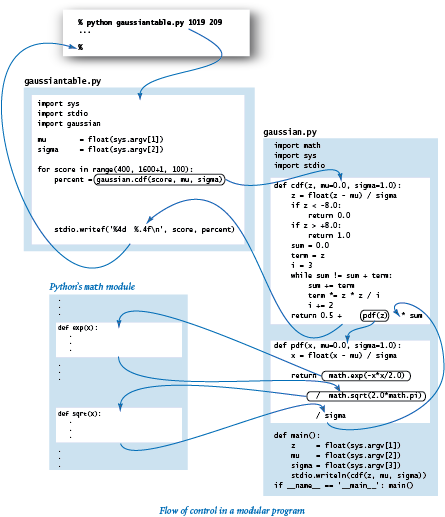### Modular programming.

The potential effect of programming by defining multiple files, each an independent module with multiple functions, is another profound change in our programming style. Generally, we refer to this approach as modular programming. The key benefit of modular programming is that encourages us to break a computation up into smaller parts that can be individually debugged and tested. Generally, you should compose every program by identifying a reasonable way to divide the computation into separate parts of a manageable size and implement each part as if someone will want to use it later.

## Modular Programming Abstractions

Next, we describe abstractions that serve as the basis of modular programming.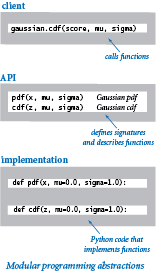### Implementations.

We use the generic term implementation to describe code that implements a set of functions that are intended for reuse. A Python module is an implementation: we refer to the set of functions collectively with a name module and keep them in a file module.py. The guiding principle in module design is to provide to clients the functions that they need and no others.

### Clients.

We use the generic term client to refer to a program that makes use of an implementation. We say that a Python program (a script or a module) that calls a function that is defined in a file named `module.py` is a client of `module`.

### Application programming interfaces (APIs).

Programmers normally think in terms of a contract between the client and the implementation that is a clear specification of what the implementation is to do. You have been able to compose programs that are clients of `math` and `random` and other standard Python modules because of an informal contract (an English-language description of what they are supposed to do) along with a precise specification of the signatures of the functions that are available for use. Collectively, this information is known as an application programming interface (API). The same mechanism is effective for user-defined modules. The API allows any client to use the module without having to examine the code that defines the module. When we compose a new module, we always provide an API. For example, this is the API for our gaussian.py module:How much information should an API contain? In this booksite, we stick to a principle that parallels our guiding design principle: provide to client programmers the information they need and no more.

### Private functions.

Sometimes we wish to define in a module a helper function that is not intended to be called directly by clients. We refer to such a function as a private function. By convention, Python programmers use an underscore as the first character in the name of a private function. For example, the following is an alternative implementation of the `pdf()` function from gaussian.py that calls the private function _phi():

 ```def _phi(x): return math.exp(-x*x/2.0) / math.sqrt(2*math.pi) def pdf(x, mu=0.0, sigma=1.0): return _phi(float((x - mu) / sigma)) / sigma ```

We do not include private functions in APIs because they are not part of the contract between clients and implementations. Indeed, a leading underscore in the name of a function signals clients not to call the function explicitly. (Regrettably, Python has no mechanism for enforcing this convention.)

### Libraries.

A library is a collection of related modules. For example, Python has a standard library (which includes the modules `random` and `math`) and many extension libraries (such as `NumPy` for scientific computing and `Pygame` for graphics and sound). Also, for this book, we provide a booksite library (which includes the modules `stdio` and `stddraw`).

### Documentation.

The APIs of all standard, extension, and booksite modules are available through the built-in `help()` function in interactive Python. As illustrated below, all you need to do is type `python` (to enter interactive Python), then enter the statement `import module` (to load the module), then type `help(module)` to see the API for module. The APIs of the standard and extension Python modules also are available in another form through the online Python documentation.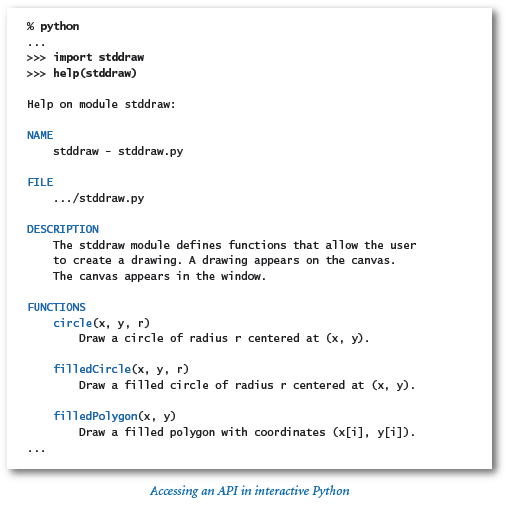Next, we present the APIs for our `stdrandom` module (for generating random numbers), our `stdarray` module (for one- and two-dimensional arrays), and our `stdstats` module (for statistical calculations). We also describe some interesting clients of these modules.

## Random Numbers

The `stdrandom.py` module is for generating random numbers from various distributions.### API design.

We make certain assumptions about the objects passed to each function in `stdrandom`. For example, we assume that clients will pass to `stdrandom.bernoulli()` a float between 0.0 and 1.0, and to `stdrandom.discrete()` an array of nonnegative numbers (not all of which are zero). Such assumptions are part of the contract between the client and the implementation.

### Unit testing.

We implement `stdrandom` without reference to any particular client, but it is good programming practice to include a basic test client `main()` that, at a minimum,

• Exercises all the code.
• Provides some assurance that the code is working.
• Takes an argument from the command line to allow flexibility in testing.

Although it is not intended for clients, we use `main()` when debugging, testing, and improving the functions in a module. This practice is call unit testing. Proper unit testing can be a significant programming challenge in itself. In this particular case, it is appropriate to do more extensive testing in a separate client to check that the numbers have many of the same properties as truly random numbers drawn from the cited distributions.

### Stress testing.

An extensively used module such as `stdrandom` should also be subject to stress testing, where we make sure that it does not fail unexpectedly, even when the client does not follow the contract or makes some assumption that is not explicitly covered. What should `stdrandom.discrete()` do if some array elements are negative? What if the argument is an array of length 0? What should `stdrandom.uniform()` do if the second argument is less than (or equal to) the first argument? Such cases are sometimes referred to as corner cases.

## Array-Processing API

In Section 1.4 we saw the utility of functions that create one-dimensional arrays of a specified length and two-dimensional arrays with a specified number of rows and columns. Thus, we introduced the `stdarray` module from the booksite library, and specifically its functions for creating and initializing arrays `stdarray.create1D()` and `stdarray.create2D()`.

Moreover, we have seen and will continue to see many examples where we wish to read values from standard input into an array and write values from an array to standard output. Accordingly, we have included in the `stdarray` module functions for reading arrays of integers, floats, and booleans from standard input and for writing them to standard output — thus complementing the `stdio` module. Here is the full API for `stdarray`: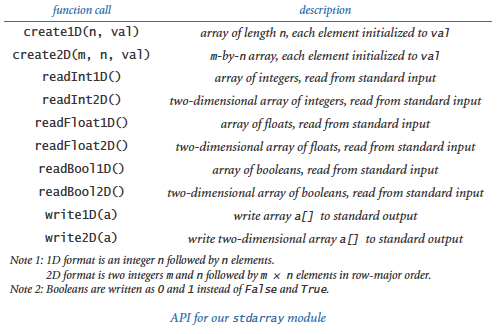We have adopted the convention that arrays appearing on standard input include the dimension(s) and appear in the order indicated, as illustrated in the diagram below. The `read*()` functions expect this format, and the `write*()` functions produce output in this format.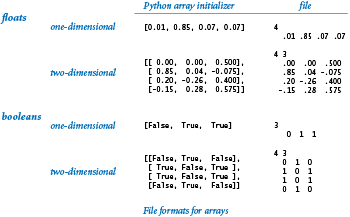For arrays of booleans, our file format uses `0` and `1` values instead of `False` and `True`. This convention is much more economical for large arrays. More important, patterns in the data are much easier to spot with this file format.

## Iterated Function Systems

An iterated function system (IFS) is a general way to produce fractals like the Sierpinski triangle and the Barnsley Fern. As a first example, consider the following simple process: start by plotting a point at one of the vertices of an equilateral triangle. Then pick one of the three vertices at random and plot a new point halfway between the point just plotted and that vertex. Continue performing the same operation.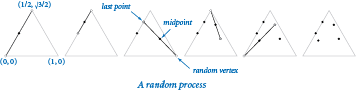The program sierpinski.py simulates this process. Below are snapshots after 1000, 10000, and 100000 steps. You might recognize the figure as the Sierpinski triangle.The program ifs.py is a data-driven version program that simulates a generalization of this process. See the textbook for details. You can run it on any of these input files: barnsley.txt, binary.txt, coral.txt, culcita.txt, cyclososus.txt, dragon.txt, fishbone.txt, floor.txt, koch.txt, sierpinski.txt, spiral.txt, swirl.txt, tree.txt, or zigzag.txt.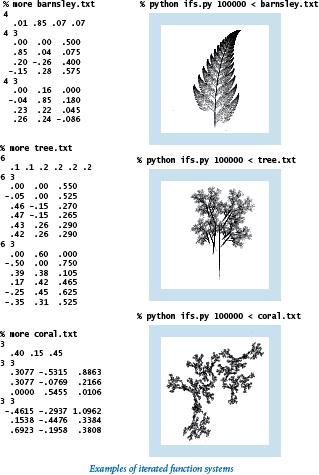The ability to produce such realistic diagrams so easily suggests intriguing scientific questions: What does computation tell us about nature? What does nature tell us about computation?

## Standard Statistics

The `stdstats.py` module is for statistical calculations and basic visualizations, as articulated in the following API. See the textbook for details.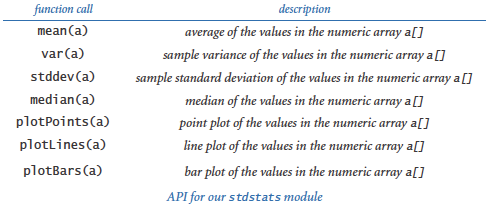### Bernoulli trials.

Program bernoulli.py is an example `stdstats.py` client. It counts the number of heads found when a fair coin is flipped n times and compares the result with the predicted Gaussian distribution function. According to the Central Limit Theorem, the resulting histogram is extremely well approximated by the Gaussian distribution with mean n/2 and variance n/4. This is the output of the command `python bernoulli.py 20 100000`:## Modular Programming Benefits

The module implementations that we have developed illustrate modular programming. Instead of composing a new program that is self-contained in its own file to address a new problem, we break up each task into smaller, more manageable subtasks, then implement and independently debug code that addresses each subtask. The ifs.py and bernoulli.py programs exemplify modular programming because they are relatively sophisticated computations that are implemented with several relatively small modules.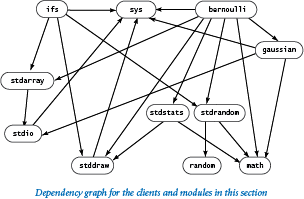We emphasize modular programming throughout this booksite because it has many important benefits, including the following:

• Programs of a reasonable size. No large task is so complex that it cannot be divided into smaller subtasks.
• Debugging. With modular programming and our guiding principle of keeping the scope of variables local to the extent possible, we severely restrict the number of possibilities that we have to consider when debugging. Equally important is the idea of a contract between client and implementation. Once we are satisfied that an implementation is meeting its end of the bargain, we can debug all its clients under that assumption.
• Code reuse. Once we have implemented libraries such as StdStats and StdRandom, we do not have to worry about writing code to compute averages or standard deviations or to generate random numbers again — we can simply reuse the code that we have written.
• Maintenance. Like a good piece of writing, a good program can always be improved. Supppose that while developing a new client, you find a bug in some module. With modular programming, fixing that bug amounts to fixing bugs in all of the module's clients.

#### Q & A

Q. How can I make a booksite module (such as `stdio` or `stdrandom`) available to my Python programs?

A. If you followed the step-by-step instructions on the booksite for installing Python, all of our standard modules (including `stdio`, `stddraw`, `stdarray`, `stdrandom`, and `stdstats`) should already be available for use in your Python programs.

Q. How can I make non-standard and non-booksite modules (such as `gaussian`) available to my Python programs?

A. Let's be specific. Suppose you've composed a program named `myprogram.py`, and `myprogram.py` imports `gaussian`. The issue is how you can make the `gaussian` module available to `myprogram.py`. In other words, the issue is how you can tell Python where to find the `gaussian.py` file while it is executing `myprogram.py`.

The easiest approach is to place `gaussian.py` in the same directory as `myprogram.py`. However, using that approach you might end up with multiple copies of `gaussian.py` — one copy in each directory that contains a `gaussian.py` client. That approach would make the `gaussian.py` code difficult to maintain.

An alternative approach is to place a single copy of `gaussian.py` in some directory, and then set the `PYTHONPATH` environment variable to contain that directory. Subsequently, Python looks for files in that directory whenever it encounters an `import` statement.

The mechanism for setting the `PYTHONPATH` environment variable depends upon the operating system that you're using. Suppose your computer is running Mac OS X or Linux. Further suppose you place `gaussian.py` in the directory `/Users/yourusername/common`. Then these commands are appropriate:

 ```export PYTHONPATH=/Users/yourusername/common python myprogram.py ```

The `export` command sets the value of the `PYTHONPATH` variable to `/Users/yourusername/common`. So during the execution of the subsequent `python myprogram.py` command, Python looks in the `/Users/yourusername/common` directory when handling `import` statements, and so finds the `gaussian.py` file.

Generalizing, on Mac OS X and Linux systems the value of the `PYTHONPATH` variable is a sequence of directories separated by colons. So after you issue this command:

 ```export PYTHONPATH=directory1:directory2:directory3 ```

Python looks in `directory1`, then `directory2`, and then `directory3` to find `.py` files when handling `import` statements.

On the other hand, suppose your computer is running Microsoft Windows. Further suppose you place `gaussian.py` in the directory `c:\Users\yourusername\common`. Then these commands are appropriate:

 ```set PYTHONPATH=c:\Users\yourusername\common python myprogram.py ```

The `set` command sets the value of the `PYTHONPATH` variable to `c:\Users\yourusername\common`. So during the execution of the subsequent `python myprogram.py` command, Python looks in the `c:\Users\yourusername\common` directory when handling `import` statements, and so finds the `gaussian.py` file.

Generalizing, on Microsoft Windows systems the value of the `PYTHONPATH` variable is a sequence of directories separated by semicolons. So after you issue this command::

 ```set PYTHONPATH=directory1;directory2;directory3 ```

Python looks in `directory1`, then `directory2`, and then `directory3` to find `.py` files when handling `import` statements.

Q. I tried to import the `gaussian` module, but got the following error message. What's wrong?

 ```ImportError: No module named gaussian ```

A. You did not make `gaussian` available to Python, as described above.

Q. I tried to call `gaussian.pdf()` but I got the following error. What's wrong?

 ```NameError: name 'gaussian' is not defined ```

A. You left out the `import gaussian` statement.

Q. Is there a keyword that identifies a `.py` file as a module (and not a script)?

A. No. Technically, the key point is to avoid arbitrary global code using the patterns described earlier in this section. If you avoid arbitrary global code in a `.py` file, so that the `.py` file can be imported into some other `.py` file, then we call it a module. Pragmatically, however, there is a bit of a conceptual leap in this viewpoint, because it is one thing to sit down to create a `.py` file that you will run (and perhaps run again sometime later with different data), but quite another thing to create a `.py` file that you will rely on much later in the future, and still another thing to create a `.py` file for someone else to use in the future.

Q. How do I develop a new version of a module that I have been using for a while?

A. With care. Any change to the API might break any client program, so it is best to work in a separate directory. Of course, with this approach you are working with a copy of the code. If you are changing a module that has a lot of clients, you can appreciate the problems faced by companies putting out new versions of their software. If you just want to add a few functions to a module, go ahead: that is usually not too dangerous.

Q. How do I know that an implementation behaves properly? Why not automatically check that it satisfies the API?

A. We use informal specifications because composing a detailed specification is not much different from composing a program. Moreover, a fundamental tenet of theoretical computer science says that doing so does not even solve the basic problem because there is no way in general to check that two different programs perform the same computation.

Q. I notice that files whose names are suffixed with `.pyc` are appearing when I run the programs from this section. For example, when I issue the command `python gaussiantable.py`, I notice that Python automatically creates a file named `gaussian.pyc`. What are these `.pyc` files?

A. As noted in Section 1.1, whenever Python executes a program, it translates the program into an internal (not human-readable) form that is more amenable to execution. That internal form is called bytecode. When you import a module for the first time, Python compiles the code and stores the resulting bytecode in a `.pyc` file. This makes the module load faster because Python does not need to recompile it each time (but it does not make running the program any faster).

When a program consists of only one file, Python discards the file's bytecode after execution is complete. However, when a program consists of multiple files — that is, when a program consists of a client file and modules — Python does not discard the bytecode that it generates for the modules. Instead it stores that bytecode in `.pyc` files.

For example, recall that gaussiantable.py depends upon gaussian.py. So when you issue the command `python gaussiantable.py 1019 209`, Python translates gaussiantable.py into bytecode. It also translates gaussian.py into bytecode. Eventually it discards the former; but it saves the latter in the file `gaussian.pyc`.

Python's rationale is this: Since gaussian.py was used as a module in this program, it's likely that it will be used in the future as a module in other programs. So Python saves the bytecode version of gaussian.py in `gaussian.pyc` to avoid the translation overhead if the module indeed is reused.

It's fine to delete `.pyc` files at any time; Python will regenerate them when appropriate. It also is fine not to delete `.pyc` files because if you edit a `.py` file after Python has generated the corresponding `.pyc` file, Python will regenerate the `.pyc` file automatically.

#### Exercises

1. Compose a module that implements the hyperbolic trigonometric functions based on the definitions sinh(x) = (ex - e-x)/2 and cosh(x) = (ex + e-x)/2, with tanh(x), coth(x), sech(x), and csch(x) defined in a manner analogous to standard trigonometric functions.

2. Compose a test client for both `stdstats` and `stdrandom` that checks that all of the methods in both modules operate as expected. Take a command-line argument `n`, generate `n` random numbers using each of the functions in `stdrandom`, and write their statistics. Extra credit: Defend the results that you get by comparing them to those that are to be expected from mathematical analysis.

3. Develop a client that does stress testing for `stdrandom`. Pay particular attention to `discrete()`. For example, are the probabilities nonnegative? Are they all zero?

4. Compose a function that takes floats `ymin` and `ymax` (with `ymin` strictly less than `ymax`) and a `float` array `a[]` as arguments and linearly scales the elements in `a[]` so that they are all between `ymin` and `ymax`.

5. Compose a gaussian.py and `stdstats.py` client that explores the effects of changing the mean and standard deviation on the Gaussian distribution curve. Create one plot with curves having a fixed mean and various standard deviations and another with curves having a fixed standard deviation and various means.

6. Add to `stdrandom.py` a function `maxwellBoltzmann()` that returns a random value drawn from a Maxwell-Boltzmann distribution with parameter σ. To produce such a value, return the square root of the sum of the squares of three Gaussian random variables with mean 0 and standard deviation σ. (The speeds of molecules in an ideal gas have a Maxwell-Boltzmann distribution.)

7. Modify bernoulli.py to animate the bar graph, replotting it after each experiment, so that you can watch it converge to the normal distribution.

8. Modify bernoulli.py to add a command-line argument p that specifies the probability that a biased coin comes up heads. Run experiments to get a feeling for the distribution corresponding to a biased coin. Be sure to try values of p that are close to 0 and close to 1.

9. Compose a module `matrix.py` that implements the following API for vectors and matrices (see Section 1.4):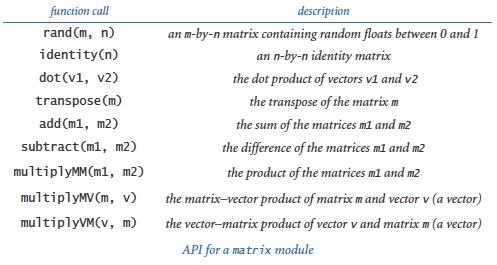Solution: See matrix.py.

10. Compose a client of `matrix.py` (from the previous exercise) which uses the following code:

 ```moves = int(sys.argv) p = stdarray.readFloat2D() ranks = stdarray.create1D(len(p), 0.0) ranks = 1.0 for i in range(moves): ranks = matrix.multiplyVM(ranks, p) stdarray.write1D(ranks) ```

That code performs the same calculation as markov.py (from section 1.6).

In practice, mathematicians and scientists use mature libraries such as `NumPy` (or special-purpose matrix-processing languages such as `Matlab`) for such tasks because they are likely to be more efficient, accurate, and robust than anything you could compose yourself. The NumPy Appendix describes how to use `NumPy`.

11. Compose a client of `matrix.py` (from the previous two exercises) named `markovsquaring.py` that implements the version of markov.py (from Section 1.6) but is based on squaring the matrix, instead of iterating the vector-matrix multiplication.

12. Redesign randomsurfer.py (from Section 1.6) using the `stdarray` and `stdrandom` modules.

Partial solution:

 ```... p = stdarray.readFloat2D() page = 0 # Start at page 0. hits = stdarray.create1D(n, 0) for i in range(moves): page = stdrandom.discrete(p[page]) hits[page] += 1 ... ```
13. Add a function `exp()` to `stdrandom.py` that takes an argument λ and returns a random number from the exponential distribution with rate λ. Hint: If x is a random number uniformly distributed between 0 and 1, then -ln x / λ is a random number from the exponential distribution with rate λ.

#### Creative Exercises

1. Sicherman dice. Suppose that you have two six-sided dice, one with faces labeled 1, 3, 4, 5, 6, and 8 and the other with faces labeled 1, 2, 2, 3, 3, and 4. Compare the probabilities of occurrence of each of the values of the sum of the dice with those for a standard pair of dice. Use `stdrandom` and `stdstats`.

Solution: Dice with these properties are called Sicherman dice: they produce sums with the same frequency as regular dice (2 with probability 1/36, 3 with probability 2/36, and so on).

2. Craps. Here are the rules for a pass bet in the game of craps: Roll two 6-sided dice, and let x be their sum.

• If x is 7 or 11, you win.
• If x is 2, 3, or 12, you lose.

Otherwise, repeatedly roll two the dice until their sum is either x or 7.

• If their sum is x, you win.
• If their sum is 7, you lose.

Compose a modular program to estimate the probability of winning a pass bet. Modify your program to handle loaded dice, where the probability of a die landing on 1 is taken from the command line, the probability of landing on 6 is 1/6 minus that probability, and 2-5 are assumed equally likely. Hint: Use `stdrandom.discrete()`.

3. Dynamic histogram. Suppose that the standard input stream is a sequence of floats. Compose a program that takes an integer `n` and two floats `l` and `r` from the command line and uses `stdstats` to plot a histogram of the count of the numbers in the standard input stream that fall in each of the `n` intervals defined by dividing `(l, r)` into `n` equal-sized intervals. Use your program to add code to your solution to Exercise 2 (from earlier in this section) to plot a histogram of the distribution of the numbers produced by each function, taking `n` from the command line.

4. Tukey plot. A Tukey plot is a data visualization that generalizes a histogram, and is appropriate for use when each integer in a given range is associated with a set of `y` values. For each integer i in the range, we compute the mean, standard deviation, 10th percentile, and 90th percentile of all the associated y-values; draw a vertical line with x-coordinate i running from the 10th percentile y value to the 90th percentile y value; and then draw a thin rectangle centered on the line that runs from one standard deviation below the mean to one standard deviation above the mean. Suppose that the standard input stream is a sequence of pairs of numbers where the first number in each pair is an int and the second a double value. Compose a `stdstats` and `stddraw` client that takes an integer `n` from the command line and, assuming that all the integers on the standard input stream are between 0 and `n-1`, uses `stddraw` to make a Tukey plot of the data.

5. IFS. Experiment with various inputs to ifs.py to create patterns of your own design like the Sierpinksi triangle, Barnsley fern, or other examples. You might begin by experimenting with minor modifications to the given inputs.

6. IFS matrix implementation. Compose a version of ifs.py that uses `matrix.multiply()` (as developed in a previous exercise in this section) instead of the equations that compute the new values of `x` and `y`.

7. Stress test. Compose a client that does stress testing for `stdstats.py`. Work with a classmate, with one person composing code and the other testing it.

8. Gamblers ruin. Compose a `stdrandom.py` client to study the gamblers ruin problem (see gambler.py from Section 1.3, and the exercises at the end of that section). Note: Defining a function for the experiment is more difficult than for bernoulli.py because a function cannot return two values. But remember that a function can return a single array that contains two values.

9. Module for properties of integers. Compose a module based on the functions that we have considered in this book for computing properties of integers. Include functions for determining whether a given integer is prime; whether two integers are relatively prime; computing all the factors of a given integer; the greatest common divisor and least common multiple of two integers; Eulers totient function (see the Euler's Totient Function exercise in Section 2.1); and any other functions that you think might be useful. Create an API, a client that performs stress testing, and clients that solve several of the exercises earlier in this book.

10. Voting machines. Compose a `stdrandom.py` client (with appropriate functions of its own) to study the following problem: Suppose that in a population of 100 million voters, 51% vote for candidate A and 49% vote for candidate B. However, the voting machines are prone to make mistakes, and 5% of the time they produce the wrong answer. Assuming the errors are made independently and at random, is a 5% error rate enough to invalidate the results of a close election? What error rate can be tolerated?

11. Poker analysis. Compose a `stdrandom.py` and `stdstats.py` client (with appropriate functions of its own) to estimate the probabilities of getting one pair, two pair, three of a kind, a full house, and a flush in a five-card poker hand via simulation. Divide your program into appropriate functions and defend your design decisions. Extra credit: Add straight and straight flush to the list of possibilities.

12. Music module. Develop a module based on the functions in playthattunedeluxe.py (from Section 2.1) that you can use to compose client programs to create and manipulate songs.

13. Animated plots. Compose a program that takes a command-line argument `m` and produces a bar graph of the `m` most recent floats on standard input. Use the same animation technique that we used for bouncingball.py (from Section 1.5): erase, redraw, show, and wait briefly. Each time your program reads a new number, it should redraw the whole graph. Since most of the picture does not change as it is redrawn slightly to the left, your program will produce the effect of a fixed-size window dynamically sliding over the input values. Use your program to plot a huge time-variant data file, such as stock prices.

14. Array plot module. Develop your own plot functions that improve upon those in `stdstats.py`. Be creative! Try to make a plotting module that you think you will use for some application in the future.